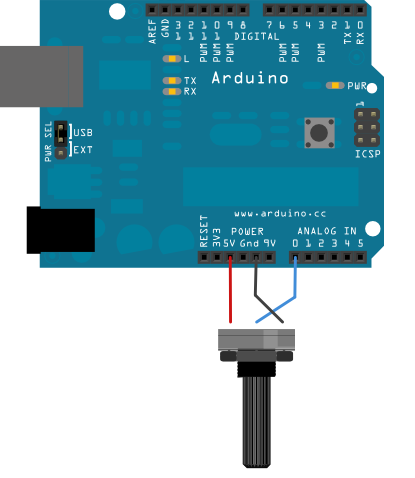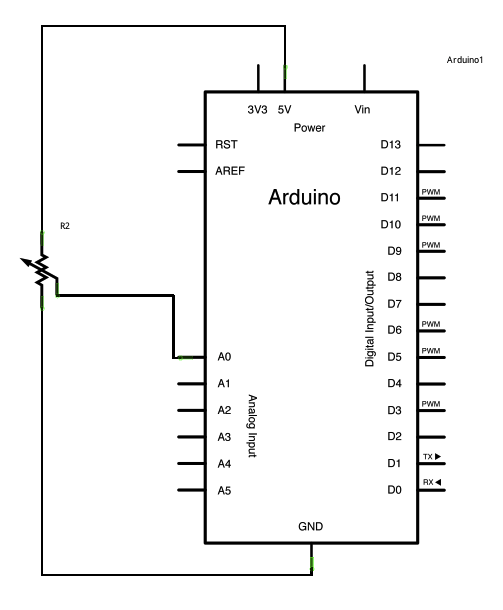## Smoothing

This sketch reads repeatedly from an analog input, calculating a running average and printing it to the computer. This example is useful for smoothing out the values from jumpy or erratic sensors, and also demonstrates the use of arrays to store data.

### Hardware

• Arduino or Genuino Board
• 10k ohm potentiometer

### Circuit

click the image to enlargeimage developed using Fritzing. For more circuit examples, see the Fritzing project page

Connect one pin of a potentiometer to 5V, the center pin to analog pin 0, and the the last pin to ground.

### Schematic

click the image to enlarge### Code

The code below sequentially stores 10 readings from your analog sensor into an arrays, one by one. With each new value, the sum of all the numbers is generated and divided, producing an average value which then be used to smooth outlying data. Because this averaging takes place each time a new value is added to the array (rather then waiting for 10 new values, for instance) there is no lag time in calculating this running average.

Altering the size of the array used, by changing `numReadings` to a larger value will smooth the data collected even further.

/*
Smoothing

Reads repeatedly from an analog input, calculating a running average and
printing it to the computer. Keeps ten readings in an array and continually
averages them.

The circuit:
- analog sensor (potentiometer will do) attached to analog input 0

created 22 Apr 2007
by David A. Mellis  <dam@mellis.org>
modified 9 Apr 2012
by Tom Igoe

This example code is in the public domain.

http://www.arduino.cc/en/Tutorial/Smoothing
*/

// Define the number of samples to keep track of. The higher the number, the
// more the readings will be smoothed, but the slower the output will respond to
// the input. Using a constant rather than a normal variable lets us use this
// value to determine the size of the readings array.
const int numReadings = 10;

int readIndex = 0;              // the index of the current reading
int total = 0;                  // the running total
int average = 0;                // the average

int inputPin = A0;

void setup() {
// initialize serial communication with computer:
Serial.begin(9600);
// initialize all the readings to 0:
}
}

void loop() {
// subtract the last reading:
// read from the sensor:
// add the reading to the total:
// advance to the next position in the array:

// if we're at the end of the array...
// ...wrap around to the beginning:
}

// calculate the average:
average = total / numReadings;
// send it to the computer as ASCII digits
Serial.println(average);
delay(1);        // delay in between reads for stability
}

• array
• if
• for
• serial

• AnalogInOutSerial - Read an analog input pin, map the result, and then use that data to dim or brighten an LED.
• AnalogInput - Use a potentiometer to control the blinking of an LED.
• AnalogWriteMega - Fade 12 LEDs on and o¬ff, one by one, using an Arduino or Genuino Mega board.
• Calibration - Define a maximum and minimum for expected analog sensor values.
• Fading - Use an analog output (PWM pin) to fade an LED.

Last revision 2015/07/29 by SM